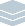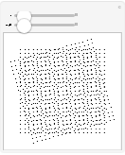Wolfram Archive
Wolfram Programming Lab is a legacy product.
All the same functionality and features, including access to Programming Lab Explorations, are available with Wolfram|One.
Start programming now. »
Try it now »
(no sign-in required)# Moiré Patterns

Overlay grids of points and see patterns emerge.

Run the code to make an array of points. Try increasing the size from 3:

This draws a grid of 9 points:

Graphics[{ {Point[{-1, -1}], Point[{-1, 0}], Point[{-1, 1}]}, {Point[{0, -1}], Point[{0, 0}], Point[{0, 1}]}, {Point[{1, -1}], Point[{1, 0}], Point[{1, 1}]} }]

An easier way to make a grid of points is with Table.

This makes a grid of points where x and y vary from -1 to 1 in steps of 1:

Table[Point[{x, y}], {x, -1, 1}, {y, -1, 1}]

This draws the grid:

Graphics[Table[Point[{x, y}], {x, -1, 1}, {y, -1, 1}]]

Put more points in the grid by changing 1 to 3:

Graphics[ Table[Point[{x, y}], {x, -3, 3}, {y, -3, 3}]]

Graphics[Table[Point[{x, y}], {x, -3, 3}, {y, -3, 3}]]

Change the size of the grid with a slider:

Make anything interactive with Manipulate.

To make the grid interactive, replace the 3s with the variable n, wrap the Graphics expression with Manipulate and specify that n varies from 3 to 10 in steps of 1. Now you can change the size of the grid by dragging the slider:

Manipulate[ Graphics[Table[Point[{x, y}], {x, -n, n}, {y, -n, n}]], {n, 3, 10, 1} ]

Manipulate[ Graphics[Table[Point[{x, y}], {x, -n, n}, {y, -n, n}]], {n, 3, 10, 1} ]

Include a second grid, rotated by 30 degrees. Try rotating by different amountsfor example, 45 degrees:

Use With to give the table of points the name points (this will make the next steps easier):

With[{points = Table[Point[{x, y}], {x, -10, 10}, {y, -10, 10}]}, Graphics[points] ]

Rotate the points by 30 degrees:

With[{points = Table[Point[{x, y}], {x, -10, 10}, {y, -10, 10}]}, Graphics[Rotate[points, 30 Degree]] ]

Draw the unrotated and rotated points together:

With[{points = Table[Point[{x, y}], {x, -10, 10}, {y, -10, 10}]}, Graphics[{points, Rotate[points, 30 Degree]}] ]

With[{points = Table[Point[{x, y}], {x, -10, 10}, {y, -10, 10}]}, Graphics[{points, Rotate[points, 30 Degree]}] ]

Allow the angle to be varied interactively:

This gives two interactive grids rotated 30 degrees from each other:

Manipulate[ With[{points = Table[Point[{x, y}], {x, -n, n}, {y, -n, n}]}, Graphics[{points, Rotate[points, 30 Degree]}] ], {n, 3, 10, 1} ]

Add an angle control by replacing 30 with the variable angle and specifying that the angle varies from 0 to 180:

Manipulate[ With[{points = Table[Point[{x, y}], {x, -n, n}, {y, -n, n}]}, Graphics[{points, Rotate[points, angle Degree]}] ], {n, 3, 10, 1}, {angle, 0, 180} ]

Manipulate[ With[{points = Table[Point[{x, y}], {x, -n, n}, {y, -n, n}]}, Graphics[{points, Rotate[points, angle Degree]}] ], {n, 3, 10, 1}, {angle, 0, 180} ]

Share ItMake an interactive website for exploring moiré patterns:

Deploy the Manipulate to the Wolfram Cloud, where anyone with a browser can use it:

CloudDeploy[ Manipulate[ With[{points = Table[Point[{x, y}], {x, -n, n}, {y, -n, n}]}, Graphics[{points, Rotate[points, angle Degree]}] ], {n, 3, 10, 1}, {angle, 0, 180} ], Permissions -> "Public" ]

Click the link in the output to visit the site.